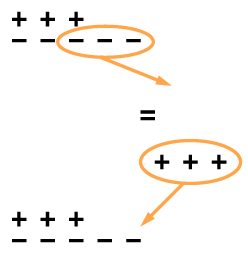### Home > CC2 > Chapter 3 > Lesson 3.2.2 > Problem3-46

3-46.

Find the value of each expression below. Change any subtraction problem to an equivalent addition problem. Draw a diagram with $+$ and $-$ tiles to justify your answer. Homework Help ✎

1. $5-7$

The equivalent addition problem is $5+(-7)$.

1. $-5+(-7)$

The drawing should look something like this:
$-----$
$-------$

1. $-5+7$

$2$
1. $-5-(-7)$
For the expression $-2-(-3)$, a drawing like this might help you to write your equivalent addition problem: